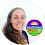## Pages

### Polynomial Long Division in Algebra 2

A while back I shared a reference sheet for students learning how to divide polynomials using synthetic division. We focus on this method in our Algebra 2 class, but because polynomial long division is in the standards, I thought to also make a reference sheet to help students with this method.

In the example on the cheat sheet, students are guided through the steps needed to find the imaginary zeros of a given polynomial function.

Students start at the "start" arrow with step 1.

"What's missing to make x multiply to get x^3?"

Oh! 2 x's!

So an x^2 goes up top. Students then multiply this x^2 by x+2, subtract, bring down and start the process over again.
To complete the process, students use the Quadratic Formula on x^2 - 4x + 5 to find the two complex zeros of the original polynomial.

1.1.